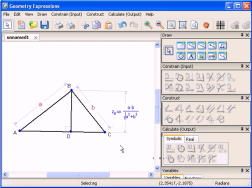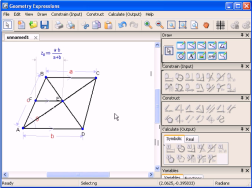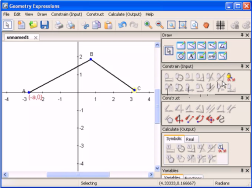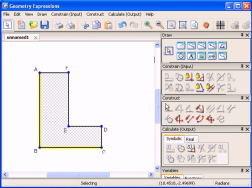# Geometry Expressions

## Getting Started

Videos illustrating many of the features of Geometry Expressions### Altitude of a Right Triangle

A simple example to demonstrate Geometry Expression's constraint based approach to geometry and its symbolic output capability.### Trapezoid

Geometry Expressions finds a surprisingly simple equation for distance from the midpoint of a trapezoid's side to its center.### Ellipse as a Locus

Here we demonstrate the usual string based construction of an ellipse foci (-a,0) (a,0).### Area of a Polygon

This example demonstrates how Geometry Expressions calculates area using either numbers or variables.